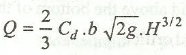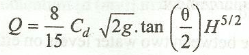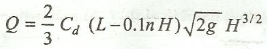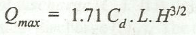Important Expressions used in Notches and Weirs

The following are the important expressions used in notches and weirs.

(a) Discharge over a rectangular notch or weir,where
b = Width of notch or weir, and
H = Height of liquid above the sill of the notch.

(b) Discharge over a triangular notch or weir,For a right angled V-notch, Theeta = 90°.

(c) Discharge over a trapezoidal notch or weir (also called Cippoletti weir) is equal to the sum of discharge over a rectangular notch or weir and the discharge over a triangular notch or weir.

(d) Discharge over a rectangular weir, according to Francis formula is given bywhere
n = Number of end contractions.

(e) Maximum discharge over a broad crested weir,Notes:
1. A weir is said to be a broad crested weir, if the width of the crest of the weir is more than half the height of water above the weir crest.
2. A weir is said to be a narrow crested weir, if the width of the crest of the weir is less than half the height of water above the weir crest.
3. When the water level on the downstream side of a weir is above the top surface of a weir, then the weir is known as submerged or drowned weir.
4. A weir, generally, used as a spillway of a dam is Ogee weir.
5. It has been observed that whenever water is flowing over a rectangular weir, having no end contractions, the nappe (i.e., the sheet of water flowing over the weir) touches the side walls of the channel. After flowing over the weir, the nappe falls away from the weir, thus creating a space beneath the water. In such a case, some air is trapped beneath the weir.
6. If the atmospheric pressure exists beneath the nappe, it is then known as free nappe.
7. If the pressure below the nappe is negative, it is then called a depressed nappe.
8. Sometimes, no air is left below the water and the nappe adheres or clings to the downstream side of the weir. Such a nappe is called clinging nappe or an adhering nappe.